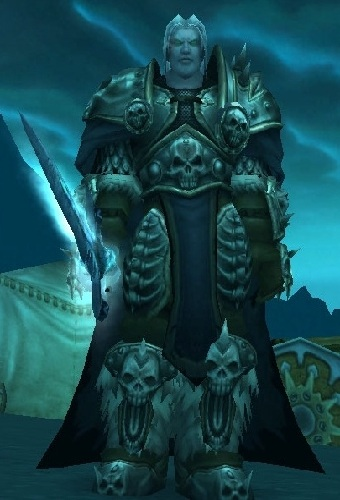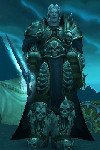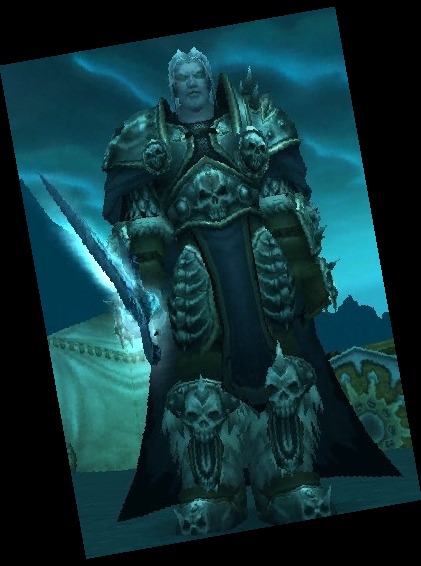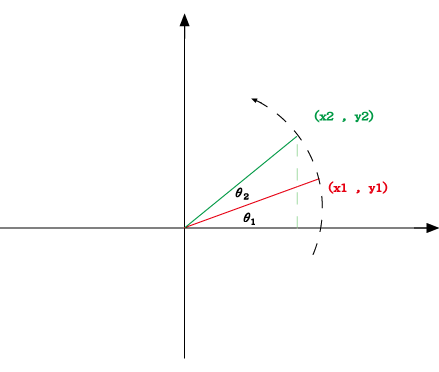2012
Aug
02

# Teajs GD library tutorial

Tags:

GD Extension 主要功能是可以對圖片做處理，簡單的有圖片縮小、放大、切割，也可以用來製作圖片，這篇文章將會實做圖片的縮小，旋轉與銳利化。

## Teajs GD Extension 安裝

g++ -shared -lgd -lpthread -lv8 -lrt -ldl -o gd.so ~/gd.o ../binary/bytestorage.os ../../common.os ../../app.os ../../path.os ../../cache.os

## Javascript GD 畫圖!

TrueColor 是一種由 32 bits 存儲的圖片格式，在三原色 RGB 中各用了 8個 bits ，每一個顏色可以存的數值是 0~255 ，這樣就有 2563 種組合，另外再配上「透明度 alpha」8 bits。

『jpeg』 使用的格式是 TrueColor , 『gif』使用的格式則是 Palette ，因此 gif 只有 256 種顏色可以使用，『png』則支援 TrueColor 與 Palette。

## 縮圖前的阿蕯斯## 縮圖後的阿蕯斯## Resize 程式範例

Resize.sjs
`var Image = require('gd').Image;var source = "Arthas-H.jpg";var img = resize(source , 100,150);img.save(Image.PNG , "tmp.png"); function resize(img ,to_w,to_h) {    var pos = img.lastIndexOf('.');    var ext = img.substring(pos+1, img.length);    var type = Image.JPEG;    switch(ext.toLowerCase()) {        case 'jpeg':case 'jpg':            type = Image.JPEG;            break;         case 'png':            type = Image.PNG;            break;         case 'gif':            type = Image.GIF;            break;      }    var source_img = new Image(type , img);    var w = source_img.sx();    var h = source_img.sy();    var image = new Image(Image.TRUECOLOR, to_w, to_h);    var destx=0,desty=0;    var srcx=0,srcy=0;    image = image.copyResized(source_img, destx, desty, srcx, srcy, to_w, to_h , w , h );    return image;} `

## 旋轉前的阿蕯斯## 旋轉後的阿蕯斯## Rotate 旋轉原理

# 數學上定義的旋轉，是指用座標軸逆時針旋轉 => 座標點為順時針旋轉 ，所以算出的結果有一丁點不同。• 圖中可以算半徑 z = x12+y12 的根號
• x2 = z × cos(θ1+θ2) = z×(cosθ1×cosθ2 - sinθ1×sinθ2)
• 因為 cosθ1 = x1/z , sinθ1 = y1/z
• 得 x2 = x1×cosθ2 - y1×sinθ2
• 一樣的算法可以求得 y2 = y1×cosθ2 + x1×sinθ2

## Rotate 圖片旋轉程式範例

Rotate.sjs
`var source = "Arthas-H.jpg";var img = rotate(source , 10);img.save(Image.PNG , "tmp.png"); function rotate(img , angle) {    var PI = Math.PI;    var radian = PI*angle/180;    var pos = img.lastIndexOf('.');    var ext = img.substring(pos+1, img.length);    var type = Image.JPEG;     switch(ext.toLowerCase()) {        case 'jpeg':case 'jpg':            type = Image.JPEG;            break;         case 'png':            type = Image.PNG;            break;         case 'gif':            type = Image.GIF;            break;    }    var source_img = new Image(type , img);    var w = source_img.sx();    var h = source_img.sy();    var p2x = w/2, p2y = h/2;    var diffw = Math.abs((p2x*Math.cos(radian) + p2y*Math.sin(radian))) - Math.abs(p2x);    diffw = 2* Math.abs(diffw);    var diffh = Math.abs(( -p2x*Math.sin(radian) + p2y*Math.cos(radian))) - Math.abs(p2y);    diffh = 2* Math.abs(diffh);     var centerx = Math.round(w/2),centery = Math.round(h/2);    var newH = h + diffh;    var newW = w + diffw;    var image = new Image(Image.TRUECOLOR, newW, newH);    var destx=newW/2,desty=newH/2;//rotate in center    var srcx=0,srcy=0;    image = image.copyRotated(source_img, destx, desty, srcx, srcy, w, h , angle );    return image;} `

## 正常的阿蕯斯## 銳利化後的阿蕯斯## 銳利化程式範例

sharpen.sjs
`var img = sharpen(source, 150);img.save(Image.PNG , "tmp.png");function sharpen(img , percent) {    var pos = img.lastIndexOf('.');    var ext = img.substring(pos+1, img.length);    var type = Image.JPEG;     switch(ext.toLowerCase()) {        case 'jpeg':case 'jpg':            type = Image.JPEG;            break;         case 'png':            type = Image.PNG;            break;         case 'gif':            type = Image.GIF;            break;    }     var source_img = new Image(type , img);     var data = source_img.sharpen(percent);    return data;} `